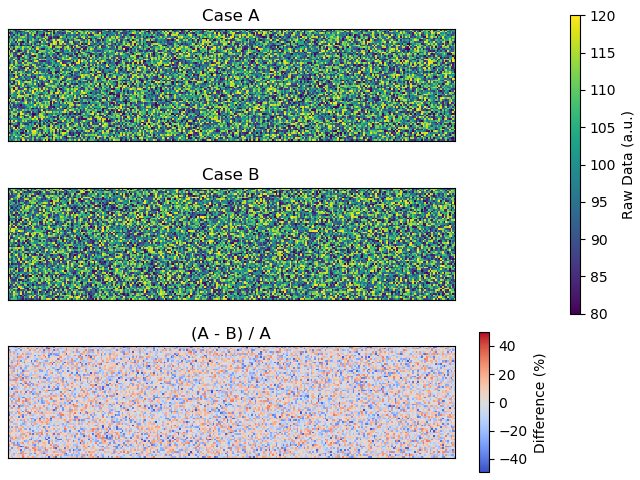# Surprising alignment of (multiple) colorbars with constrained layout active #10627

Closed
opened this issue Feb 28, 2018 · 2 comments

Projects
None yet
Contributor

### Bug report

Bug summary

The new experimental layout manager seems to produce a rather surprising alignment of two colorbars when one is shared by several `Axes` instances, and another one is simply stealing space from a single `Axes` instance. It seems like @jklymak might have a fix in mind, but he also have a day-job ^^: milestoning for 2.2 just in case he manages to have a shot in time for it.

NB: originally from Gitter.

Code for reproduction

```import matplotlib.pyplot as plt
import numpy as np

# Dummy data

arr_shape = (64, 256)

vmin, vmax = 80.0, 120.0
arr_A = np.random.uniform(low=vmin, high=vmax, size=arr_shape)
arr_B = np.random.uniform(low=vmin, high=vmax, size=arr_shape)

rel_error = 100 * (arr_A - arr_B) / arr_A

# Plotting time!

fig, axs = plt.subplots(3, 1, constrained_layout=True)

# Raw data arrays, with a shared colorbar
for ax, arr, lbl in ((axs, arr_A, "Case A"), (axs, arr_B, "Case B")):
_im0 = ax.imshow(arr, vmin=vmin, vmax=vmax)
ax.set_title(lbl)
fig.colorbar(_im0, ax=axs[:2], label="Raw Data (a.u.)", aspect=2*20, pad=-12)

# Relative difference at the bottom, with its own colorbar
ax = axs
_im1 = ax.imshow(rel_error, cmap="coolwarm",
vmin=min(rel_error.min(), -rel_error.max()),
vmax=max(rel_error.max(), -rel_error.min()))
ax.set_title("(A - B) / A")
fig.colorbar(_im1, ax=axs, label="Difference (%)", aspect=20)

# Cosmeticks
for ax in axs.flat:
ax.set_xticks([])
ax.set_yticks([])

plt.show()```

@jklymak I know, I know, it is not really “minimal”, but I was originally planning to show a use case rather than a MWE. And at least, it is a standalone example 😈.

Actual outcomeExpected outcome

Workaround
The figure in the Expected outcome section was produced by passing the supplementary parameter “pad=-12” in the relevant line:

`fig.colorbar(_im0, ax=axs[:2], label="Raw Data (a.u.)", aspect=2*20, pad=-12)`

Matplotlib version

• Operating system: Linux (Fedora 27)
• Matplotlib version: 2.2.0rc1 from conda
• Python version: 3.6 from conda

Edit: English

Contributor

### jklymak commented Feb 28, 2018 • edited

 Thanks @afvincent That is not good. OTOH, there is a bit of an API problem in how to not make it happen. What is happening here: If you specify only one axes as the parent (your lowest axes), constrained_layout attaches the colorbar to the axes, inside the subplotspec. If you specify a colorbar for multiple axes (your upper two), it attaches the colorbar to the gridspec, and shrinks all the subplotspec boxes of the gridspec to make room. In your case, the bottom axes and colorbar are all inside the subplotbox for that axes, and the other colorbar is outside all the subplot boxes. So, constrained_layout is doing what I told it to! And we need this for the case where you have (for instance) two columns of axes, and attach a colorbar to an axes in the left column, then you don't want that colorbar to the right of all the subplots in the gridspec, you want it with the axes. I'd propose as a solution that we pass a gridspec-aware colorbar as an itterable so: `fig.colorbar(_im0, ax=[axs])` instead of `fig.colorbar(_im0, ax=axs)` would get you what you want. Right now all do is check that there is one element in the argument. Thats a bit obscure, but at least it'd stop playing with padding...

Merged